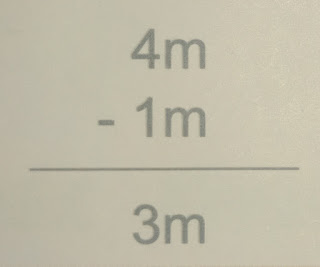# Lesson Planning of Units of Lengths

Lesson Planning of Addition and Subtraction of Units of Lengths

Subject Mathematics

Students` Learning Outcomes

• Add and subtract expressions involving similar units of lengths.
• Use appropriate units to measure lengths of different objects.
• Solve real life problems involving conversions, addition and subtraction of units of lengths.

Information for Teachers

Milometer, meter, centimeter and millimetre are units of measurement written as km, m, cm and mm respectively.Material / Resources

Writing board, chalk/marker, duster, scale, measuring tape, textbook

Introduction

• Write on the board kilometres, meters, centimetres and millimetres.
• Ask the students which unit can be used for measuring the following: as;
• Distance between cities (kilometres)
• Length of cloth (meters)
• Length of pencil (Centimetres)
• Thickness of finger nail (millimetres)
• Tell them that we have learnt basic units of length in previous lectures. Today we will learn how to add and subtract these units.
• Ask them can we add millimetres into kilometres? (Expected answer would be as; no) because only similar units can be added or subtracted.
• We make different units similar before adding and subtracting.

Development

 Activity 1 Ask the class how many units can you see written on a scale? Expected answer would be as; inch, centimeter and millimetre). Observe how many centimetres does it contain? Expected answer would be as; 30 cm) If I have 4 such scale how many centimetres they will make altogether? 1 scale = 30 cm 4 scale = 30 cm + 30 cm + 30 cm + 30 cm = 120 cmActivity 2 “Zenith went to a tailor and gave him 4 meters cloth to stitch her shirt. Tailor returned 1 meter cloth to her after stitching shirt. How much cloth is used in her shirt? Solve on the board with the help of subtraction, as;Ask them do you all wear same size of uniform? Expected answer would be as; no). Tell them that usually the distances are measured in kilometres. Whereas, the length are measured in meters and centimetres and even very small lengths are measured in millimetres. Give examples, as; Distance from Bahawalpur to Lahore = 425 km The length of bundle of cloth = 26 m The length of a needle to stitch clothes = 1=2 mm Call the name of different things from the classroom and ask the students at random which unit they will use to measure each one of them.

Sum up / Conclusion

• Same units can be added or subtracted only.
• If we want to add them we first convert them in same size.
• The smallest unit of measuring length is millimetres.
• The greatest unit for measuring length, height or distance is kilometres.
• We multiply while converting greater to smaller unit and use division when we convert smaller to greater unit.

Assessment

• Give the following problem to the students to assess them: as;
• Distance between Bahawalpur to Lahore is 425 km. if the driver has covered 291 km. how many kilometres are left to be covered?

• Choose the appropriate units for measuring the following lengths: as;
• Length of shoes:

i)                    Meter        (b) centimetres  (c) millimetres

• Widths of an eraser: as;

i)                    Millimetre (b) meters           (c) kilometres

• Assign the following questions to the students, as;